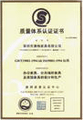China Measuring Tool & Instrument Manufacturer Based in Guilin - Tel: +86 773 5869135

### What Customer Say# How to use and read a vernier caliper

How to read the vernier calipers?

Step 1
Review the Vernier caliper's parts. Notice how it has movable jaws and two measuring scales. One of the measuring scales moves with the jaws. Use the large jaws to measure objects from the outside; these jaws point to you as you read the caliper. Use the smaller jaws to measure holes and internal spaces, such as the inside of a pipe. These jaws point away from you as you read the caliper. See each tick mark on the main ruler as one unit of measurement; for example, 2 tick marks to the right of the "0" on the main ruler reads as "2.0." Two tick marks to the right of "10" on the main ruler reads as "12.2." Read the ticks on the Vernier ruler as 1 unit of measurement on the Vernier ruler. Place a decimal point before that number when adding it to the main ruler's measurement.

Step 2
Calibrate the Vernier caliper by matching the main ruler's zero mark to the Vernier ruler's zero mark. Close the caliper's jaws completely to see if the two zero marks align with each other. If it's practical, adjust the Vernier if the zero doesn't match the zero on the main ruler. Loosen the bolt tightening it to the movable jaw, adjust the Vernier ruler so that its zero mark is perfectly aligned with the main ruler's zero mark, then tighten the bolt again.

Step 3
If calibrating the caliper is impractical, record the measuring error. Measure the distance between the Vernier's and main rulers zero marks. Know that you have a positive error if the Vernier ruler's zero is ahead of the main ruler's zero. Subtract this difference from the final measurement to get the actual object's measurement. Know that you have a negative error if the Vernier ruler's zero is to the left of the main ruler's zero. Add this difference to the final measurement.

Step 4
Place the jaws around the object you're measuring, then look at the Vernier ruler's final resting place. Look for the tick mark on the Vernier ruler that lines up perfectly to a tick on the main ruler. Use the Vernier's zero tick to determine how many ticks on the main ruler you'll factor in.

Step 5
Read the numbers on the caliper; start from the largest whole number on the main ruler to the left of the Vernier's zero mark. Count the number of whole ticks on the main ruler between the largest whole number and the Vernier's zero mark, and the tick on the Vernier that perfectly lines up to a tick on the main ruler. Add the "tick numbers" to the right of the main ruler's largest whole number; and to the left of the Vernier's zero mark. Write this number down, then place a decimal after it. Write down the number of the Vernier tick mark that perfectly aligns with the main ruler's tick mark. Place this number to the right of the decimal number (see Tips).

Tips & Warnings
For Step 5, imagine that you've just measured an object with a Vernier caliper; it's a millimeter caliper. Your Vernier's zero mark lies between the 2nd and 3rd main ruler tick to the right of the main ruler's 20.0 mm mark. You notice that the number 4 tick mark on the Vernier ruler is perfectly lined up with the 30.0 mm mark on the main ruler. Simply take 20.00 mm, add the two ticks, which are 1 mm apart, as 2 mm to get a total measurement of 22.00 mm. Since the Vernier's number 4 tick mark is perfectly lined up to one of the main ruler's tick, add .4 mm to 22 to get a total of 22.4 mm.